Atmos. Chem. Phys., 20, 3091–3105, 2020
https://doi.org/10.5194/acp-20-3091-2020
Atmos. Chem. Phys., 20, 3091–3105, 2020
https://doi.org/10.5194/acp-20-3091-2020

Research article 16 Mar 2020

Research article | 16 Mar 2020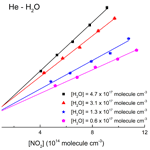# Kinetics of the OH + NO2 reaction: effect of water vapour and new parameterization for global modelling

Kinetics of the OH + NO2 reaction: effect of water vapour and new parameterization for global modelling
Damien Amedro, Matias Berasategui, Arne J. C. Bunkan, Andrea Pozzer, Jos Lelieveld, and John N. Crowley Damien Amedro et al.
• Department of Atmospheric Chemistry, Max Planck Institute for Chemistry, 55128 Mainz, Germany

Correspondence: John N. Crowley (john.crowley@mpic.de)

Abstract

The effect of water vapour on the rate coefficient for the atmospherically important, termolecular reaction between OH and NO2 was determined in He–H2O (277, 291, and 332 K) and N2H2O bath gases (292 K). Combining pulsed-laser photolytic generation of OH and its detection by laser-induced fluorescence (PLP-LIF) with in situ, optical measurement of both NO2 and H2O, we were able to show that (in contrast to previous investigations) the presence of H2O increases the rate coefficient significantly. We derive a rate coefficient for H2O bath gas at the low-pressure limit (${k}_{\mathrm{0}}^{{\mathrm{H}}_{\mathrm{2}}\mathrm{O}}$) of $\mathrm{15.9}×{\mathrm{10}}^{-\mathrm{30}}$ cm6 molecule−2 s−1. This indicates that H2O is a more efficient collisional quencher (by a factor of ≈6) of the initially formed HO–NO2 association complex than N2, and it is a factor of ≈8 more efficient than O2. Ignoring the effect of water vapour will lead to an underestimation of the rate coefficient by up to 15 %, e.g. in the tropical boundary layer. Combining the new experimental results from this study with those from our previous paper in which we report rate coefficients obtained in N2 and O2 bath gases (Amedro et al., 2019), we derive a new parameterization for atmospheric modelling of the OH +NO2 reaction and use this in a chemical transport model (EMAC) to examine the impact of the new data on the global distribution of NO2, HNO3, and OH. Use of the new parameters (rather than those given in the IUPAC and NASA evaluations) results in significant changes in the HNO3∕NO2 ratio and NOx concentrations (the sign of which depends on which evaluation is used as reference). The model predicts the presence of HOONO (formed along with HNO3 in the title reaction) in concentrations similar to those of HO2NO2 at the tropical tropopause.

Share
1 Introduction

In our recent study of the title reaction (Amedro et al., 2019), we reported extensive measurements of the rate constant (k1) for the termolecular reaction between OH and NO2 (Reaction R1) in N2 and O2 bath gas over a large range of temperatures and pressures.

$\begin{array}{}\text{(R1a)}& \mathrm{OH}+{\mathrm{NO}}_{\mathrm{2}}+\mathrm{M}& \to {\mathrm{HNO}}_{\mathrm{3}}+\mathrm{M},\text{(R1b)}& & \to \mathrm{HOONO}+\mathrm{M}.\end{array}$

Reaction (R1) converts NO2 to nitric acid (HNO3) and peroxynitrous acid (HOONO), and its rate strongly influences the relative abundance of atmospheric NOx (NO2+ NO) and longer-lived “reservoirs” of NOx, which include, for example, HNO3 and organic nitrates. It also converts OH (the main initiator of atmospheric oxidation) to a long-lived reservoir (HNO3). As the abundances of OH and NOx directly impact on photochemical ozone formation and the lifetimes of greenhouse gases, Reaction (R1) may be considered one of the most important gas-phase processes in atmospheric science (Newsome and Evans, 2017). As outlined by Amedro et al. (2019), the rate coefficients and product branching for this reaction are dependent on pressure and temperature and also on the bath-gas identity, i.e. the identity of the collision partner (M in Reaction R1). The efficiency per collision of energy transfer from the initially “hot” association complex to bath gas can vary considerably, with more complex bath gases possessing more degrees of freedom and bonds with similar vibrational frequencies to those in the association complex being generally more efficient. In this sense, we may expect H2O to be better than N2 or O2 in quenching [HO–NO2]#.

In this second part of our study of the reaction between OH and NO2, we extend the experiments to H2O and He bath gases. After N2 (≈78 %) and O2 (≈21 %), water vapour is the third most abundant gaseous species in the lower atmosphere. Its concentration is highly variable in time and space, varying in mixing ratio from a few percent at sea level to parts-per-million levels in the stratosphere. Most of the atmosphere's water vapour is present in the planetary boundary layer where its average mixing ratio on the global scale is ≈1 % but which may exceed 5 % in tropical regions.

The effect of water vapour on gas-phase radical reactions has been the subject of numerous studies (Buszek et al., 2011) and is sometimes interpreted in terms of formation of H2O–radical complexes leading, via a chaperone-type mechanism, to an increase in the rate constant. An important example of this is the HO2 self-reaction for which the rate constant increases by a factor of up to 2 in the presence of water vapour due to formation of an HO2H2O complex (Lii et al., 1981; Kircher and Sander, 1984). Theoretical calculations (Allodi et al., 2006; Sadanaga et al., 2006; Thomsen et al., 2012) suggest that, under our experimental conditions, the fraction of OH and NO2 clustered with H2O is <0.1 %, which is insufficient to significantly impact on k1.

On the other hand, the role of H2O as a collision partner in termolecular, atmospheric reactions has rarely been reported though its potential impact has been highlighted (Troe, 2003). Indeed, water vapour is known to be a more efficient third-body collider, by up to an order of magnitude, compared to N2 in termolecular reactions such as H + H + M, H + OH + M, and H +O2+ M (Getzinger and Blair, 1969; Michael et al., 2002; Fernandes et al., 2008; Shao et al., 2019).

The conclusions of three previous experiments examining the role of H2O in kinetic studies of Reaction (R1) are highly divergent, with the addition of H2O found to (1) increase the rate coefficient (Simonaitis and Heicklen, 1972), (2) have no measurable effect (D'Ottone et al., 2001), or (3) even reduce it (Sadanaga et al., 2006). The overall aim of this research was to clarify these differences and provide quantitative data on the third-body efficiency of H2O for the title reaction. Based on the kinetic data for the water-vapour effect reported in this paper and in N2 and O2 presented in the first part of this study (Amedro et al., 2019), we have generated a new parameterization for the overall rate coefficient, k1, and examined its impact on atmospheric OH, NOx, and NOy in a global chemical transport model.

2 Experimental details

The details of the experimental set-up have been published previously (Wollenhaupt et al., 2000; Amedro et al., 2019), and only a brief description is given here.

## 2.1 PLP-LIF technique

The experiments were carried out in a quartz reactor of 500 cm3 volume, which was thermostatted to the desired temperature by circulating a 60:40 mixture of ethylene glycol–water. The pressure in the reactor was monitored with 100 and 1000 Torr capacitance manometers. Flow rates were chosen so that a fresh gas sample was available for photolysis at each laser pulse (laser frequency, 10 Hz), thus preventing build-up of products. Pulses of 248 nm laser light (≈20 ns) for OH generation from HNO3, H2O2, and O3∕H2O precursors were provided by an excimer laser (Compex 205 F, Coherent) operated using KrF.

$\begin{array}{}\text{(R2)}& & {\mathrm{HNO}}_{\mathrm{3}}+h\mathit{\nu }\left(\mathrm{248}\phantom{\rule{0.125em}{0ex}}\mathrm{nm}\right)\to \mathrm{OH}+{\mathrm{NO}}_{\mathrm{2}},\text{(R3)}& & {{\mathrm{H}}_{\mathrm{2}}\mathrm{O}}_{\mathrm{2}}+h\mathit{\nu }\left(\mathrm{248}\phantom{\rule{0.125em}{0ex}}\mathrm{nm}\right)\to \mathrm{2}\mathrm{OH},\text{(R4)}& & {\mathrm{O}}_{\mathrm{3}}+h\mathit{\nu }\left(\mathrm{248}\phantom{\rule{0.125em}{0ex}}\mathrm{nm}\right)\to \mathrm{O}\left({}^{\mathrm{1}}\mathrm{D}\right)+{\mathrm{O}}_{\mathrm{2}},\text{(R5)}& & \mathrm{O}\left({}^{\mathrm{1}}\mathrm{D}\right)+{\mathrm{H}}_{\mathrm{2}}\mathrm{O}\to \mathrm{2}\mathrm{OH}.\end{array}$

OH concentrations (1011 to 1012 molecule cm−3) were similar to those reported by Amedro et al. (2019) and the same arguments, which rule out a significant influence of secondary reactions, apply. The concentration ranges of the H2O2, HNO3, and O3 precursors are listed in the notes to Tables 1 and 2.

Table 1Measurements of k1 in He bath gas.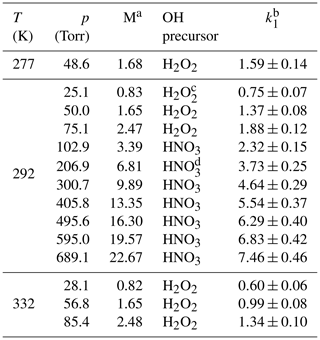a Molecular density M(He) in units of 1018 molecule cm−3. b Units of 10−12 cm3 molecule−1 s−1. The errors are 2σ total uncertainty. c Concentration range of H2O2≈514×1013 molecule cm−3. d Concentration range of HNO3≈59×1013 molecule cm−3.

Table 2Measurements of k1 in N2H2O and He–H2O bath gases.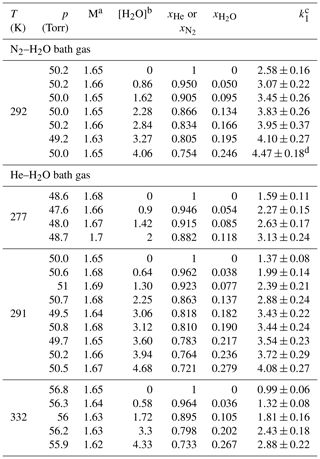Unless otherwise indicated, the measurements were performed using H2O2 as OH precursor. The concentration range of H2O2 was 5–18×1013 molecule cm−3 for experiments in He–H2O bath gas and 9–14×1013 molecule cm−3 for experiments in N2H2O bath gas. a Molecular density M(He–H2O) or M(N2H2O) in units of 1018 molecule cm−3. b Units of 1017 molecule cm−3. c Units of 10−12 cm3 molecule−1 s−1. Errors are 2σ total uncertainty. d Measurement performed using O3H2O as OH precursor (with $\left[{\mathrm{O}}_{\mathrm{3}}\right]=\mathrm{2}×{\mathrm{10}}^{\mathrm{13}}$ molecule cm−3).

OH was detected following excitation of the OH ${A}^{\mathrm{2}}\mathrm{\Sigma }\left({v}^{\prime }=\mathrm{1}\right)←{X}^{\mathrm{2}}\mathrm{\Pi }\left({v}^{\prime \prime }=\mathrm{0}\right)$ transition (Q11(1) at 281.997 nm using a YAG-pumped dye laser (Quantel Brilliant B and Lambda-Physik Scanmate)). OH fluorescence was detected by a photomultiplier tube (PMT) screened by a 309 nm interference filter and a BG 26 glass cut-off filter.

## 2.2 Online absorption measurement of NO2 and H2O concentration

As discussed by Amedro et al. (2019), the determination of the NO2 concentration is critical for accurate measurement of k1. We therefore deployed in situ, broadband (405–440 nm), and single-wavelength (365 nm) optical absorption spectroscopy techniques. The former was located prior (in flow) to the quartz reactor; the latter was located behind the quartz reactor. Using the broadband cell, the NO2 concentration was retrieved by least square fitting from 405 to 440 nm to a reference spectrum (Vandaele et al., 2002) and degraded to the resolution of our spectrometer. Simultaneously, we measured NO2 at 365 nm using the absorption cross section of $\mathrm{5.89}×{\mathrm{10}}^{-\mathrm{19}}$ cm2 molecule−1, which was determined previously by Amedro et al. (2019), who give a detailed description of the NO2 concentration measurements and the choice of reference spectrum. For the temperatures used in this study, corrections to the NO2 concentration due to formation of the N2O4 dimer were not necessary.

For the present experiments, a third absorption cell (l=40 cm) was placed downstream of the quartz reactor to measure the H2O concentration at 184.95 nm. This set-up used a low-pressure mercury Pen-Ray lamp isolated with a 185 nm interference filter as light source. Optical extinction was converted to concentrations using a cross section of $\mathrm{7.14}×{\mathrm{10}}^{-\mathrm{20}}$ cm2 molecule−1 (Cantrell et al., 1997).

## 2.3 Chemicals

N2 and He (Westfalen, 99.999 %) were used without further purification. H2O2 (AppliChem, 50 wt %) was concentrated to >90 wt % by vacuum distillation. Anhydrous nitric acid was prepared by mixing KNO3 (Sigma Aldrich, 99 %) and H2SO4 (Roth, 98 %) and condensing HNO3 vapour into a liquid-nitrogen trap. NO (Air Liquide, 3.5) was purified of other nitrogen oxides by fractional vacuum distillation and then converted to NO2 via reaction with a large excess of O2. The NO2 thus made was trapped in liquid N2, and the excess O2 was pumped out. The resulting NO2 was stored as a mixture of ∼0.5 % NO2 in N2 or ∼5.5 % NO2 in He. Distilled H2O (Merck, liquid chromatography grade) was degassed before use and kept at constant temperature.

3 Results and discussion

## 3.1 Measurements of k1 in He bath gas and comparison with literature

Our study of the role of H2O as collision partner in Reaction (R1) was carried out in mixtures of He–H2O and N2H2O. In order to separate the effects of H2O and He, we also required accurate rate coefficients for pure He bath gas, which we describe below. As for the N2 and O2 bath-gas datasets (Amedro et al., 2019), the experiments were carried out under pseudo-first-order conditions ([NO2]  [OH]) so that Eqs. (1)–(2) describe the decay of OH and the derivation of the bimolecular rate coefficient k1.

$\begin{array}{}\text{(1)}& \left[\mathrm{OH}{\right]}_{t}=\left[\mathrm{OH}{\right]}_{\mathrm{0}}\mathrm{exp}\left(-{k}^{\prime }t\right),\end{array}$

where [OH]t is the concentration (molecule cm−3) at time t after the laser pulse. k is the pseudo-first-order rate coefficient and is defined as

$\begin{array}{}\text{(2)}& {k}^{\prime }={k}_{\mathrm{1}}\left[{\mathrm{NO}}_{\mathrm{2}}\right]+{k}_{d},\end{array}$

where kd (s−1) accounts for OH loss due to diffusion out of the reaction zone and reaction with its photolytic precursors such as HNO3 or H2O2.

An exemplary dataset illustrating OH decays and a plot of k versus [NO2] is given in Fig. S1 of the Supplement.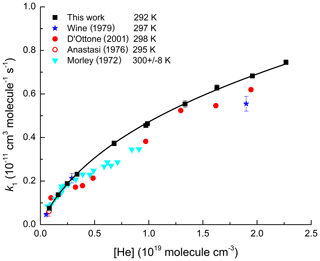Figure 1Values of k1 from this study (black squares) as a function of He concentration at 292 K. Errors are 2σ statistical only. The solid line is a fit to our data using Eq. (4) with ${k}_{\mathrm{0}}=\mathrm{1.4}×{\mathrm{10}}^{-\mathrm{30}}$ cm6 molecule−2 s−1, ${k}_{\mathrm{\infty }}=\mathrm{6.3}×{\mathrm{10}}^{-\mathrm{11}}$ cm3 molecule−1 s−1, Fc=0.32, m=3.1, and n=0. Previous datasets at room temperature (Wine et al., 1979; D'Ottone et al., 2001; Anastasi and Smith; 1976; Morley and Smith, 1972) are displayed for comparison.

Values of k1 obtained in He bath gas (25–690 Torr, 292 K) are summarized in Figs. 1 and 2 and listed in Table 1. The kinetics of termolecular reactions can be described by the Lindemann–Hinshelwood mechanism, whereby the rate constant at the low-pressure limit (k0, units of cm6 molecule−2 s−1) is proportional to the pressure, and at the high-pressure limit (k, units of cm3 molecule−1 s−1) is independent of pressure. In the intermediate pressure range, the fall-off regime, the rate coefficient is a function of both low-pressure (k0) and high-pressure (k) rate coefficients and the (reaction-partner-dependent) broadening factor F, which accounts for the lower rate constant measured in the fall-off regime than predicted by the Lindemann–Hinshelwood mechanism reactions (Troe, 1983). Under the conditions of temperature and pressure relevant for atmospheric chemistry, the title reaction is in the fall-off regime.

$\begin{array}{}\text{(3)}& k=\frac{{k}_{\mathrm{0}}\left[M\right]{k}_{\mathrm{\infty }}}{{k}_{\mathrm{0}}\left[M\right]+{k}_{\mathrm{\infty }}}F\end{array}$

The solid lines in Figs. 1 and 2 are fits according to the Troe formalism for termolecular reactions (Troe, 1983) as adopted by the IUPAC panel in their evaluation of atmospheric reactions:

$\begin{array}{}\text{(4)}& k\left(PT\right)=\frac{{k}_{\mathrm{0}}{\left(\frac{T}{\mathrm{300}}\right)}^{-m}\left[\mathrm{M}\right]{k}_{\mathrm{\infty }}{\left(\frac{T}{\mathrm{300}}\right)}^{-n}}{{k}_{\mathrm{0}}{\left(\frac{T}{\mathrm{300}}\right)}^{-m}\left[\mathrm{M}\right]+{k}_{\mathrm{\infty }}{\left(\frac{T}{\mathrm{300}}\right)}^{-n}}F,\end{array}$

where T is the temperature in kelvin, [M] is the bath-gas concentration (molecule cm−3), and m and n are dimensionless temperature exponents.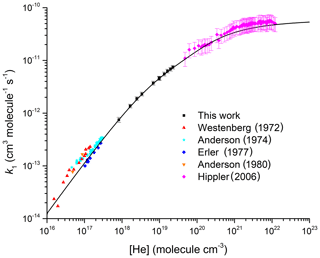Figure 2Comparison between the present dataset; the high-pressure measurements by Hippler et al. (2006); and the low-pressure measurements by Anderson et al. (1974), Westenberg and Dehaas (1972), Anderson (1980), and Erler et al. (1977). All measurements were made at room temperature. The black line is our parameterization with ${k}_{\mathrm{0}}=\mathrm{1.4}×{\mathrm{10}}^{-\mathrm{30}}$ cm6 molecule−2 s−1, ${k}_{\mathrm{\infty }}=\mathrm{6.3}×{\mathrm{10}}^{-\mathrm{11}}$ cm3 molecule−1 s−1, m=3.1, n=0, and Fc=0.32.

$\begin{array}{}\text{(5)}& \mathrm{log}F=\frac{\mathrm{log}{F}_{\mathrm{c}}}{\mathrm{1}+{\left[\mathrm{log}\left(\frac{{k}_{\mathrm{0}}{\left(\frac{T}{\mathrm{300}}\right)}^{-m}\left[M\right]}{{k}_{\mathrm{\infty }}{\left(\frac{T}{\mathrm{300}}\right)}^{-n}}\right)/N\right]}^{\mathrm{2}}},\end{array}$

where N=(0.75–1.27 log Fc) and Fc is the broadening factor at the centre of the fall-off curve.

As discussed in some detail in the first part of our studies of the title reaction (Amedro et al., 2019), the low- or high-pressure rate constants for the title reaction (k0 and k) are not well defined by existing datasets, which do not deliver sufficiently accurate rate coefficient at very low pressures (<1 mbar) or at very high pressures (>500 bar). Studies in which k has been derived from rates of vibrational relaxation of OH (Smith and Williams, 1985; D'Ottone et al., 2005) return values of k that provide some constraint on its value, but the associated uncertainty is too large to consider this parameter well defined.

In our previous paper, Amedro et al. (2019) describe highly accurate measurements of k1 over a wide range of temperatures and pressures in the fall-off regime. From measurements of k1 in N2 bath gas, we retrieved values for k0 and k of $\mathrm{2.6}×{\mathrm{10}}^{-\mathrm{30}}$ cm6 molecule−2 s−1 and $\mathrm{6.3}×{\mathrm{10}}^{-\mathrm{11}}$ cm3 molecule−1 s−1, respectively, by fixing Fc to a value of 0.39, which has a theoretical basis (Cobos and Troe, 2003). The reasons for choosing this value of Fc are discussed in Amedro et al. (2019). Note that whereas k0 is dependent on the bath gas used, at the high-pressure limit, k should be the same in N2, O2, He, or H2O bath gases.

In Fig. 1 we display pressure-dependent rate coefficients (solid, black squares) obtained in He bath gas at 292 K. The black line is a fit (Eq. 4) to our data with k fixed to $\mathrm{6.3}×{\mathrm{10}}^{-\mathrm{11}}$ cm3 molecule−1 s−1 and n=0 as derived from an extensive dataset obtained using N2 bath gas (Amedro et al., 2019). For this dataset, the best fit is obtained with Fc=0.32, and ${k}_{\mathrm{0}}^{\mathrm{He}}=\mathrm{1.4}×{\mathrm{10}}^{-\mathrm{30}}$ cm6 molecule−2 s−1. When using Fc=0.39 (i.e. same value as that obtained in N2 bath gas), the fit slightly overestimates (∼5 %) the measurements at pressures above ∼300 Torr, whereas it underestimates by 10 % at lower pressures (Fig. S2). We note that using a higher Fc=0.39 resulted in a lower value of ${k}_{\mathrm{0}}^{\mathrm{He}}$ equal to $\mathrm{1.0}×{\mathrm{10}}^{-\mathrm{30}}$ cm6 molecule−2 s−1. The T-dependence factor in He, m(He), was determined to be 3.1 over the temperature range from 277 to 332 K (Table 1 and Fig. S6).

The high precision of our measurements in He and N2 indicates that different broadening factors (Fc) are required to interpret the pressure dependence of k1 obtained in N2 and He. This can be rationalized by considering that Fc is the product of strong-collision (${F}_{\mathrm{c}}^{\mathrm{SC}}$) and weak-collision (${F}_{\mathrm{c}}^{\mathrm{WC}}$) components (Eqs. 6–8) (Gilbert et al., 1983; Troe, 1983; Troe and Ushakov, 2011):

$\begin{array}{}\text{(6)}& & {F}_{\mathrm{c}}\approx {F}_{\mathrm{c}}^{\mathrm{SC}}{F}_{\mathrm{c}}^{\mathrm{WC}},\text{(7)}& & {F}_{\mathrm{c}}^{\mathrm{SC}}\approx {S}_{\mathrm{K}}^{-\mathrm{0.62}}\approx {\left(\mathrm{1}+\frac{r}{\mathrm{2}}\right)}^{-\mathrm{0.62}},\text{(8)}& & {F}_{\mathrm{c}}^{\mathrm{WC}}\approx {\mathit{\beta }}_{\mathrm{c}}^{\mathrm{0.14}}.\end{array}$

Here, SK is the Kassel parameter, r is the total number of external rotational modes of the reactants (equal to 5 in the reaction between OH and NO2), and βc is the collision efficiency. While the strong collision component is independent of bath gas (${F}_{\mathrm{c}}^{\mathrm{SC}}\approx \mathrm{0.46}$ for the title reaction) a change in ${F}_{\mathrm{c}}^{\mathrm{WC}}$ due to a lower collision efficiency (βc) of He relative to N2 is likely.

The collision efficiency for N2, which was used to calculate Fc=0.39, was βc(N2)≈0.3 (Troe, 2001). The value of Fc=0.32 from our He data implies βc(He)≈0.08, a factor of 3.7 times lower than βc(N2). A large difference in collision efficiency between N2 and He is consistent with theoretical calculations (Glänzer and Troe, 1974; Troe, 2001; Golden et al., 2003).

In Fig. 1, we also compare our measurements of k1 in He with data collected in the same pressure range using similar techniques. The three first measurements (Morley and Smith, 1972; Anastasi and Smith, 1976; Wine et al., 1979) used flash photolysis of H2O as a OH precursor with detection of OH by resonance fluorescence. Morley and Smith (1972) reported rate coefficients at pressures of 20 to 280 Torr at room temperature with the NO2 concentration calculated manometrically. Our parametrization agrees within the combined uncertainty of both measurements (Fig. S3). Anastasi and Smith (1976) reported one value of k1 at 25 Torr of He, which is ≈20 % lower than our measurement. Wine et al. (1979) presented values of k1 at three pressures of He. The agreement with our parameterization at the lowest two pressures is excellent but a deviation of ≈20 % is observed at the highest pressure (Fig. S4). As both studies measured NO2 concentrations using optical absorption at 365 nm, the ≈20 % difference is significant. Most recently, D'Ottone et al. (2001) reported rate coefficients from 30 to 600 Torr of He using a very similar approach to ours, i.e. PLP-LIF technique with in situ measurements of NO2 by absorption at 365 nm. The disagreement (up to 40 %) between our measurements and theirs exceed the combined reported uncertainty (Fig. S5). While it is unclear what could have caused the discrepancy, we note that the data of D'Ottone et al. (2001) are significantly more scattered and do not describe a smooth increase in rate coefficient with pressure as expected from termolecular reactions in the fall-off regime. This would appear to indicate an underestimation of the total uncertainty in their study.

Figure 2 extends the pressure range to additionally display data obtained in low-pressure flow tubes (Westenberg and Dehaas, 1972; Anderson et al., 1974; Erler et al., 1977; Anderson, 1980) and the high-pressure measurements by Hippler et al. (2006). At low pressures our data are in excellent agreement (within 10 %) with the data of Erler et al. (1977), but they predict values ≈40 % lower than those reported by Westenberg and Dehaas (1972) and Anderson (1980). The data of Anderson et al. (1974) display a large intercept ($\mathrm{4.9}×{\mathrm{10}}^{-\mathrm{14}}$ cm3 molecule−1 s−1) at zero pressure, which is attributed to a second-order heterogeneous removal rate constant. As indicated in a critical assessment of the low-pressure data by Amedro et al. (2019), it is unclear whether one can simply subtract a constant value equal to the intercept (obtained from a linear fit) to each data point. If we were to do so, the work by Anderson et al. (1974) would be in very good agreement with the low-pressure study by Erler et al. (1977) as well as with our parameterization extended to low pressures. Additionally, Amedro et al. (2019) demonstrated that, owing to the large asymmetric broadening of fall-off for this reaction, the assumption that the rate coefficient is in the low-pressure limit at N2 pressures of 0.5 Torr <p<10 Torr is invalid and leads to underestimation of k0. This observation is still true of datasets obtained at low pressures of He, so that while very good agreement is observed between our parameterization and individual rate coefficients obtained between 3 and 8 Torr of He, reported values of ${k}_{\mathrm{0}}^{\mathrm{He}}$ are 40 % lower than our values obtained from the fall-off analysis. As indicated in Fig. 2, our parameterization of k1 in He is in very good agreement with the high-pressure data reported by Hippler et al. (2006).

## 3.2 Influence of H2O on k1

As mentioned above, the effect of water vapour on k1 was determined in mixtures of H2O with both N2 and He. This is because the vapour pressure of H2O at room temperature (≈17 Torr at 293 K) is too low to enable experiments in pure H2O bath gas to be conducted using our instrument. The measurements were performed at low density ([M] $=\mathrm{1.6}×{\mathrm{10}}^{\mathrm{18}}$ molecule cm−3; 50 Torr at 293 K) where the relative increase in k1 in the presence of H2O is pronounced, resulting in greater accuracy in the determination of ${k}_{\mathrm{0}}^{{\mathrm{H}}_{\mathrm{2}}\mathrm{O}}$. Experimental data on the influence of H2O on k1 were obtained in N2H2O and He–H2O mixtures by varying the H2O mixing ratio, ${x}_{{\mathrm{H}}_{\mathrm{2}}\mathrm{O}}$, from 0.05 to 0.27 ([H2O]=0.94.5×1017 molecule cm−3) while keeping the total pressure constant at 50 Torr. Under these conditions, the addition of H2O resulted in an increase in k1 up to a factor of 2 as illustrated by the datasets of Fig. 3 in which the increase in slope as more water vapour is added is proportional to the increase in k1 (Eq. 2). At the highest concentration of water vapour (4.5×1017 molecule cm−3) the rate coefficient in He–H2O increased by a factor of >3 compared to that obtained in pure He (see Table 1).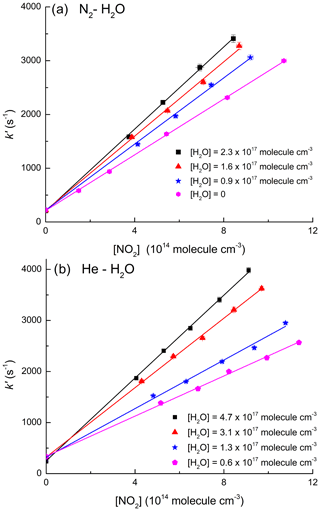Figure 3(a) Data obtained in N2H2O bath gas (50 Torr, 292 K). (b) Data obtained in He–H2O bath gas (50 Torr, 291 K). Both panels display first-order OH decay constants in various concentrations of NO2 and different mole fractions of H2O. The solid lines represent least squares linear fits to Eq. (2).

In order to determine the temperature dependence of the enhancement in k1 caused by the presence of water, the experiments in He were carried out at three different temperatures (277, 291, and 332 K). The values of k1 obtained from these experiments are plotted versus the mole fraction of H2O in Fig. 4b. At the pressures used in our experiments, k1 displays fall-off, precluding a direct measurement of ${k}_{\mathrm{0}}^{{\mathrm{H}}_{\mathrm{2}}\mathrm{O}}$.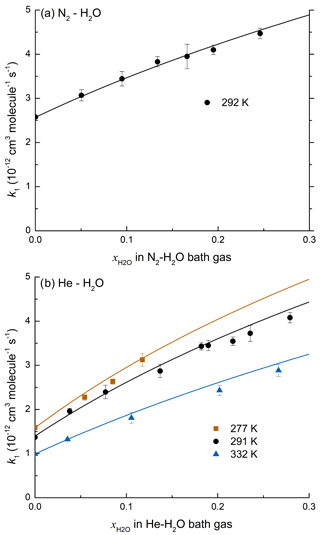Figure 4(a) k1 as a function of ${x}_{{\mathrm{H}}_{\mathrm{2}}\mathrm{O}}$ at 50 Torr N2H2O and 292 K. The line represents a least squares multivariate fit (Eqs. 7 and 8) with ${k}_{\mathrm{\infty }}=\mathrm{6.3}×{\mathrm{10}}^{-\mathrm{11}}$ cm3 molecule−2 s−1, ${k}_{\mathrm{0}}^{{\mathrm{N}}_{\mathrm{2}}}=\mathrm{2.6}×{\mathrm{10}}^{-\mathrm{30}}$ cm6 molecule−2 s−1, Fc=0.39, m=3.6, ${k}_{\mathrm{0}}^{{\mathrm{H}}_{\mathrm{2}}\mathrm{O}}=\mathrm{15.9}×{\mathrm{10}}^{-\mathrm{30}}$ cm6 molecule−2 s−1, and o=3.4. (b) k1 as a function of ${x}_{{\mathrm{H}}_{\mathrm{2}}\mathrm{O}}$ in He–H2O mixtures at 277, 291, and 332 K. The solid lines represent a least squares multivariate fit (Eqs. 7 and 9 to 12) where ${k}_{\mathrm{\infty }}=\mathrm{6.3}×{\mathrm{10}}^{-\mathrm{11}}$ cm3 molecule−2 s−1, ${k}_{\mathrm{0}}^{\mathrm{He}}=\mathrm{1.4}×{\mathrm{10}}^{-\mathrm{30}}$ cm6 molecule−2 s−1, ${F}_{\mathrm{c}}^{\mathrm{He}}=\mathrm{0.32}$, m=3.1, ${k}_{\mathrm{0}}^{{\mathrm{H}}_{\mathrm{2}}\mathrm{O}}=\mathrm{15.9}×{\mathrm{10}}^{-\mathrm{30}}$ cm6 molecule−2 s−1, ${F}_{\mathrm{c}}^{{\mathrm{H}}_{\mathrm{2}}\mathrm{O}}=\mathrm{0.39}$, and o=3.4.

The total rate constant measured in, for example, a H2ON2 bath gas is not equal to the sum of the individual rate constants calculated from the mixing ratios of N2 and H2O; i.e. ${k}_{{\mathrm{N}}_{\mathrm{2}}-{\mathrm{H}}_{\mathrm{2}}\mathrm{O}}\ne {k}_{{\mathrm{N}}_{\mathrm{2}}}+{k}_{{\mathrm{H}}_{\mathrm{2}}\mathrm{O}}$; ${k}_{{\mathrm{N}}_{\mathrm{2}}-{\mathrm{H}}_{\mathrm{2}}\mathrm{O}}$ is only equal to the sum of ${k}_{{\mathrm{N}}_{\mathrm{2}}}$ and ${k}_{{\mathrm{H}}_{\mathrm{2}}\mathrm{O}}$ at the low-pressure limit (≪1 Torr in the case of the OH reaction with NO2) and under certain conditions where gas mixtures are composed of strong colliders and/or have similar collision efficiencies (Troe, 1980; Burke and Song, 2017). Additionally, at the high-pressure end of the fall-off curve, the rate coefficient is independent of bath gas composition. To be able to make a reasonable prediction of this effect under atmospheric conditions, where the mole fraction of water vapour (${x}_{{\mathrm{H}}_{\mathrm{2}}\mathrm{O}}$) can be as large as 0.05, we analysed our measurements using two different approaches to determine ${k}_{\mathrm{0}}^{{\mathrm{H}}_{\mathrm{2}}\mathrm{O}}$. In the first case, the low-pressure rate constant in a N2H2O mixture is defined as the sum of two individual low-pressure-limit rate constants,

$\begin{array}{}\text{(9)}& \begin{array}{rl}& k\left(p,T\right)=\\ & \frac{\left({x}_{{\mathrm{N}}_{\mathrm{2}}}{k}_{\mathrm{0}}^{{\mathrm{N}}_{\mathrm{2}}}{\left(\frac{T}{\mathrm{300}}\right)}^{-m}+{x}_{{\mathrm{H}}_{\mathrm{2}}\mathrm{O}}{k}_{\mathrm{0}}^{{\mathrm{H}}_{\mathrm{2}}\mathrm{O}}{\left(\frac{T}{\mathrm{300}}\right)}^{-o}\right)\left[M\right]{k}_{\mathrm{\infty }}{\left(\frac{T}{\mathrm{300}}\right)}^{-n}}{\left({x}_{{\mathrm{N}}_{\mathrm{2}}}{k}_{\mathrm{0}}^{{\mathrm{N}}_{\mathrm{2}}}{\left(\frac{T}{\mathrm{300}}\right)}^{-m}+{x}_{{\mathrm{H}}_{\mathrm{2}}\mathrm{O}}{k}_{\mathrm{0}}^{{\mathrm{H}}_{\mathrm{2}}\mathrm{O}}{\left(\frac{T}{\mathrm{300}}\right)}^{-o}\right)\left[M\right]+{k}_{\mathrm{\infty }}{\left(\frac{T}{\mathrm{300}}\right)}^{-n}}F,\end{array}\end{array}$

where ${x}_{{\mathrm{N}}_{\mathrm{2}}}$ and ${x}_{{\mathrm{H}}_{\mathrm{2}}\mathrm{O}}$ are the mixing ratio for N2 and H2O, respectively; ${k}_{\mathrm{0}}^{{\mathrm{N}}_{\mathrm{2}}}$ and ${k}_{\mathrm{0}}^{{\mathrm{H}}_{\mathrm{2}}\mathrm{O}}$ are low-pressure-limiting rate constants (units of cm6 molecule−2 s−1) for pure N2 and H2O; k is the high-pressure limit rate constant (units of cm3 molecule−1 s−1); T is the temperature in kelvin; [M] is the molecular density (molecule cm−3); and m, n, and o are dimensionless temperature exponents.

$\begin{array}{}\text{(10)}& \begin{array}{rl}& \mathrm{log}F=\\ & \frac{\mathrm{log}{F}_{\mathrm{c}}}{\mathrm{1}+{\left[\mathrm{log}\left(\frac{\left({x}_{{\mathrm{N}}_{\mathrm{2}}}{k}_{\mathrm{0}}^{{\mathrm{N}}_{\mathrm{2}}}{\left(\frac{T}{\mathrm{300}}\right)}^{-m}+{x}_{{\mathrm{H}}_{\mathrm{2}}\mathrm{O}}{k}_{\mathrm{0}}^{{\mathrm{H}}_{\mathrm{2}}\mathrm{O}}{\left(\frac{T}{\mathrm{300}}\right)}^{-o}\right)\left[M\right]}{{k}_{\mathrm{\infty }}{\left(\frac{T}{\mathrm{300}}\right)}^{-n}}\right)/N\right]}^{\mathrm{2}}},\end{array}\end{array}$

where N=(0.75–1.27log Fc) and Fc is the broadening factor at the centre of the fall-off curve.

In the second approach, we follow Burke and Song (2017), where, in addition to the low-pressure limiting rate coefficients, the broadening factors for each bath gas are also mixed linearly and $\mathrm{log}{F}^{{\mathrm{N}}_{\mathrm{2}}-{\mathrm{H}}_{\mathrm{2}}\mathrm{O}}$ is defined as

$\begin{array}{}\text{(11)}& \mathrm{log}{F}^{{\mathrm{N}}_{\mathrm{2}}-{\mathrm{H}}_{\mathrm{2}}\mathrm{O}}={X}_{{\mathrm{N}}_{\mathrm{2}}}\mathrm{log}{F}^{{\mathrm{N}}_{\mathrm{2}}}+{X}_{{\mathrm{H}}_{\mathrm{2}}\mathrm{O}}\mathrm{log}{F}^{{\mathrm{H}}_{\mathrm{2}}\mathrm{O}},\end{array}$

where

$\begin{array}{}\text{(12)}& \begin{array}{rl}& {X}_{{\mathrm{N}}_{\mathrm{2}}}=\frac{{x}_{{\mathrm{N}}_{\mathrm{2}}}{k}_{\mathrm{0}}^{{\mathrm{N}}_{\mathrm{2}}}{\left(\frac{T}{\mathrm{300}}\right)}^{-m}\left[\mathrm{M}\right]}{\left({x}_{{\mathrm{N}}_{\mathrm{2}}}{k}_{\mathrm{0}}^{{\mathrm{N}}_{\mathrm{2}}}{\left(\frac{T}{\mathrm{300}}\right)}^{-m}+{x}_{{\mathrm{H}}_{\mathrm{2}}\mathrm{O}}{k}_{\mathrm{0}}^{{\mathrm{H}}_{\mathrm{2}}\mathrm{O}}{\left(\frac{T}{\mathrm{300}}\right)}^{-o}\right)\left[M\right]},\\ & {X}_{{\mathrm{H}}_{\mathrm{2}}\mathrm{O}}=\frac{{x}_{{\mathrm{H}}_{\mathrm{2}}\mathrm{O}}{k}_{\mathrm{0}}^{{\mathrm{H}}_{\mathrm{2}}\mathrm{O}}{\left(\frac{T}{\mathrm{300}}\right)}^{-m}\left[M\right]}{\left({x}_{{\mathrm{N}}_{\mathrm{2}}}{k}_{\mathrm{0}}^{{\mathrm{N}}_{\mathrm{2}}}{\left(\frac{T}{\mathrm{300}}\right)}^{-m}+{x}_{{\mathrm{H}}_{\mathrm{2}}\mathrm{O}}{k}_{\mathrm{0}}^{{\mathrm{H}}_{\mathrm{2}}\mathrm{O}}{\left(\frac{T}{\mathrm{300}}\right)}^{-o}\right)\left[M\right]}\end{array};\text{(13)}& \begin{array}{rl}& \mathrm{log}{F}^{{\mathrm{N}}_{\mathrm{2}}}=\\ & \frac{\mathrm{log}{F}_{\mathrm{c}}^{{\mathrm{N}}_{\mathrm{2}}}}{\mathrm{1}+{\left[\mathrm{log}\left(\frac{\left({x}_{{\mathrm{N}}_{\mathrm{2}}}{k}_{\mathrm{0}}^{{\mathrm{N}}_{\mathrm{2}}}{\left(\frac{T}{\mathrm{300}}\right)}^{-m}+{x}_{{\mathrm{H}}_{\mathrm{2}}\mathrm{O}}{k}_{\mathrm{0}}^{{\mathrm{H}}_{\mathrm{2}}\mathrm{O}}{\left(\frac{T}{\mathrm{300}}\right)}^{-o}\right)\left[M\right]}{{k}_{\mathrm{\infty }}{\left(\frac{T}{\mathrm{300}}\right)}^{-n}}\right)/\left(\mathrm{0.75}-\mathrm{1.27}\mathrm{log}{F}_{\mathrm{c}}^{{\mathrm{N}}_{\mathrm{2}}}\right)\right]}^{\mathrm{2}}}\end{array};\text{(14)}& \begin{array}{rl}& \mathrm{log}{F}^{{\mathrm{H}}_{\mathrm{2}}\mathrm{O}}=\\ & \frac{\mathrm{log}{F}_{\mathrm{c}}^{{\mathrm{H}}_{\mathrm{2}}\mathrm{O}}}{\mathrm{1}+{\left[\mathrm{log}\left(\frac{\left({x}_{{\mathrm{N}}_{\mathrm{2}}}{k}_{\mathrm{0}}^{{\mathrm{N}}_{\mathrm{2}}}{\left(\frac{T}{\mathrm{300}}\right)}^{-m}+{x}_{{\mathrm{H}}_{\mathrm{2}}\mathrm{O}}{k}_{\mathrm{0}}^{{\mathrm{H}}_{\mathrm{2}}\mathrm{O}}{\left(\frac{T}{\mathrm{300}}\right)}^{-o}\right)\left[M\right]}{{k}_{\mathrm{\infty }}{\left(\frac{T}{\mathrm{300}}\right)}^{-n}}\right)/\left(\mathrm{0.75}-\mathrm{1.27}\mathrm{log}{F}_{\mathrm{c}}^{{\mathrm{H}}_{\mathrm{2}}\mathrm{O}}\right)\right]}^{\mathrm{2}}};\end{array}\end{array}$

where ${F}_{\mathrm{c}}^{{\mathrm{N}}_{\mathrm{2}}}$ and ${F}_{\mathrm{c}}^{{\mathrm{H}}_{\mathrm{2}}\mathrm{O}}$ are the broadening factors at the centre of the fall-off curve for N2 and H2O.

In the case where two bath gases have identical (or very similar) values of Fc, the two approaches result in identical predictions and the first approach will be preferred for its simplicity. This is the case for N2 and H2O bath gases. However, when two bath gases have significantly different values of Fc (as is the case for He–H2O mixtures; see below) the second approach provides a more accurate parameterization.

### 3.2.1 Parameterization of k1 from data obtained in N2–H2O and He–H2O bath gases

Values of k1 obtained in N2H2O and He–H2O bath gases are listed in Table 2. Each rate coefficient obtained in N2H2O bath gas was defined by five parameters: the mixing ratio of N2 and H2O (${x}_{{\mathrm{N}}_{\mathrm{2}}}$ and ${x}_{{\mathrm{H}}_{\mathrm{2}}\mathrm{O}}$), the overall rate coefficient (k1), the molecular density [M], and the temperature T. We performed a multivariate fit of the N2H2O dataset with ${k}_{\mathrm{0}}^{{\mathrm{H}}_{\mathrm{2}}\mathrm{O}}$ as variable and all other parameters fixed with ${k}_{\mathrm{\infty }}=\mathrm{6.3}×{\mathrm{10}}^{-\mathrm{11}}$ cm3 molecule−1 s−1, ${k}_{\mathrm{0}}^{{\mathrm{N}}_{\mathrm{2}}}=\mathrm{2.6}×{\mathrm{10}}^{-\mathrm{30}}$ cm6 molecule−2 s−1, and m=3.6 as derived in Amedro et al. (2019); o was fixed to 3.4 (see below) and Fc was held at 0.39, making the assumption that the broadening factors at the centre of the fall-off curve for H2O and N2 were identical. The fit to the data returned ${k}_{\mathrm{0}}^{{\mathrm{H}}_{\mathrm{2}}\mathrm{O}}=\left(\mathrm{15.9}±\mathrm{0.7}\right)×{\mathrm{10}}^{-\mathrm{30}}$ cm6 molecule−2 s−1 where the uncertainty is 2σ (statistical only). The solid black line in Fig. 4a represents the parameterization for a varying fraction of H2O in N2 at a total pressure of 50 Torr using the parameters given above. Equating ${F}_{\mathrm{c}}^{{\mathrm{H}}_{\mathrm{2}}\mathrm{O}}$ and ${F}_{\mathrm{c}}^{{\mathrm{N}}_{\mathrm{2}}}$ simplifies the analysis, though it is likely that ${F}_{\mathrm{c}}^{{\mathrm{H}}_{\mathrm{2}}\mathrm{O}}>{F}_{\mathrm{c}}^{{\mathrm{N}}_{\mathrm{2}}}$ as the collision efficiency (βc) is likely to be larger for H2O than for N2. We found that the He–H2O data cannot be modelled by assuming the same Fc for both He and H2O bath gas, and the approach of Burke and Song (2017) was therefore preferred. In order to analyse the data, we fixed the following parameters: ${k}_{\mathrm{0}}^{{\mathrm{H}}_{\mathrm{2}}\mathrm{O}}=\mathrm{15.9}×{\mathrm{10}}^{-\mathrm{30}}$ cm6 molecule−2 s−1, ${F}_{\mathrm{c}}^{{\mathrm{H}}_{\mathrm{2}}\mathrm{O}}=\mathrm{0.39}$, ${F}_{\mathrm{c}}^{\mathrm{He}}=\mathrm{0.32}$, ${k}_{\mathrm{0}}^{\mathrm{He}}=\mathrm{1.4}×{\mathrm{10}}^{-\mathrm{30}}$ cm6 molecule−2 s−1, and m=3.1 to derive $o=\mathrm{3.4}±\mathrm{0.8}$ (2σ, statistical only), which describes the temperature dependence of the low-pressure limit in H2O as depicted in Fig. 4b.

There is clearly some uncertainty related to the arbitrary use of ${F}_{\mathrm{c}}^{{\mathrm{H}}_{\mathrm{2}}\mathrm{O}}=\mathrm{0.39}$. For example, if we were to analyse the data in N2H2O using ${F}_{\mathrm{c}}^{{\mathrm{H}}_{\mathrm{2}}\mathrm{O}}=\mathrm{0.6}$ and the linear mixing method, we would retrieve ${k}_{\mathrm{0}}^{{\mathrm{H}}_{\mathrm{2}}\mathrm{O}}=\mathrm{10}×{\mathrm{10}}^{-\mathrm{30}}$ cm6 molecule−2 s−1, which is ≈50 % lower than our preferred value. The effect of the different analyses can be assessed by comparing the predicted impact of H2O on k1 at 80 % relative humidity, 1000 mbar, and 313 K. If we set ${F}_{\mathrm{c}}^{{\mathrm{H}}_{\mathrm{2}}\mathrm{O}}=\mathrm{0.39}$, we predict that the effect of H2O is to increase k1 by 15 %, while choosing ${F}_{\mathrm{c}}^{{\mathrm{H}}_{\mathrm{2}}\mathrm{O}}=\mathrm{0.6}$ results in an increase of 20 %. Theoretical calculation of the relative values of Fc in N2, O2, and H2O bath gases input would be useful to reduce this uncertainty. Our data indicate a significant positive trend in k1 when adding H2O. As discussed above, more efficient energy transfer from [HO–NO2]# in collision with H2O compared to N2 is intuitive and supported by the present dataset as well as that of Simonaitis and Heicklen (1972), who derived ${k}_{\mathrm{0}}^{{\mathrm{H}}_{\mathrm{2}}\mathrm{O}}=\mathrm{11}×{\mathrm{10}}^{-\mathrm{30}}$ cm6 molecule−2 s−1. Given the complexity of the analysis, this may be considered to be in good agreement. This result is, however, not consistent with the observations of D'Ottone et al. (2001), who report no significant change in k1 in 150 Torr of He when adding either 10 or 20 Torr of H2O and is completely at odds with the conclusions of Sadanaga et al. (2006), who report a reduction in k1 (by 18 %) when adding 29.1 mbar of H2O at atmospheric pressure. If our value for ${k}_{\mathrm{0}}^{{\mathrm{H}}_{\mathrm{2}}\mathrm{O}}$ is correct, D'Ottone et al. (2001) should have seen an increase in k1 of ≈55 % and Sadanaga et al. (2006) should have observed an increase of ≈5 %.

A potential explanation for the very divergent observations of the effect of H2O is the heterogeneous loss of NO2 when adding H2O. We tested for NO2 loss in a set of experiments in which NO2 and H2O were monitored simultaneously while systematically varying the amount of H2O. Our results indicated a reduction in the concentration of NO2 by up to ≈20 % as we increased the concentration of H2O up to 4.5×1017 molecule cm−3. Unless NO2 is monitored in situ (as in our experiments), 20 % loss of NO2 would lead to a similar size reduction in the OH decay constant and thus an underestimation of the rate coefficient. A fractional loss of NO2 of this magnitude would explain why Sadanaga et al. (2006) found an apparent reduction in k1 when adding H2O.

However, the situation becomes more complex if NO2 is converted to trace gases that are reactive towards OH. For this reason, we performed an additional experiment to investigate whether NO2 was converted via reaction with H2O on surfaces to HONO and/or HNO3. Note that conversion of NO2 to HONO at low pressures (e.g. 50 Torr) would result in an increase in the OH decay constant (${k}_{\mathrm{OH}+\mathrm{HONO}}>{k}_{\mathrm{OH}+{\mathrm{NO}}_{\mathrm{2}}}$), whereas conversion of NO2 to HNO3 would result in a decrease (${k}_{\mathrm{OH}+{\mathrm{HNO}}_{\mathrm{3}}}<{k}_{\mathrm{OH}+{\mathrm{NO}}_{\mathrm{2}}}$).

In order to test for the presence of HONO, we modified the broadband absorption set-up by replacing the halogen lamp with a deuterium lamp, allowing us to detect HONO around 350 nm as well as NO2. The optical absorption of NO2 and HONO (340–380 nm) was monitored in a flow of NO2 (1.7×1015 cm−3) at 50 Torr of He in the absence and presence of H2O ($\left[{\mathrm{H}}_{\mathrm{2}}\mathrm{O}\right]=\mathrm{4.5}×{\mathrm{10}}^{\mathrm{17}}$ molecule cm−3, the maximum concentration used in this work). A depletion in NO2 of 21 % (3.7×1014 molecule cm−3) was observed when H2O was added. An analysis of the spectra with and without H2O (Fig. S7) enabled us to establish an upper limit to the HONO concentration of $\approx \mathrm{1}×{\mathrm{10}}^{\mathrm{13}}$ molecule cm−3, which would correspond to just 3 % of the NO2 lost. At this concentration, HONO does not significantly increase the loss rate of OH (<3 % using a rate coefficient for reaction of OH with HONO of $\mathrm{6.0}×{\mathrm{10}}^{-\mathrm{12}}$ cm3 molecule−1 s−1 (IUPAC, 2019). In the same experiment, we also recorded the optical density at 185 nm, where H2O, NO2, and HNO3 all absorb. Despite the large HNO3 absorption cross section at this wavelength ($\mathrm{1.6}×{\mathrm{10}}^{-\mathrm{17}}$ cm2 molecule−1; Dulitz et al., 2018) we found no evidence for HNO3 formation, indicating that the NO2 lost was not converted to gas-phase HNO3. Given its great affinity for glass in the presence of H2O, we expect that any HNO3 formed is strongly partitioned to the walls of the reactor. The tests indicate that, on the timescales of our experiments, NO2 is lost irreversibly on the humidified walls of our experiment. The maximum concentration of H2O used in this experiment, 4.5×1017 molecule cm−3, corresponds to a relative humidity of 80 % (at 292 K) so that H2O condensation is not expected.

It is difficult to establish whether our observations of significant NO2 loss can explain the result of D'Ottone et al. (2001), who did not observe an enhancement in k1. D'Ottone et al. (2001) did not state whether, in their experiments, NO2 and H2O were monitored simultaneously. Also, our observed loss of NO2 is not necessarily transferable to other studies as the heterogeneous loss of NO2 will vary from one experimental set-up to the next, as residence times and surface areas may vary substantially.

A very simple calculation serves to illustrate the role of water vapour as a third-body quencher for the title reaction. We consider, for example, the tropical boundary layer with a temperature of 30 C and a relative humidity of 80 % at a total pressure of 1 bar. The pressure of water vapour is 34 mbar, and those of O2 and N2 are then 210 and 756 mbar, respectively. A rough contribution of each quenching gas to the overall rate coefficient can be calculated from the respective low-pressure rate coefficients. For N2, O2, and H2O these are (in units of 10−30 cm3 molecule−1 s−1) 2.6, 2.0, and 15.9. Water vapour is therefore a factor of ≈8 more efficient than O2, and a factor of ≈6 more efficient than N2 as a quencher of the HO–NO2 intermediate, which is qualitatively consistent with known strong binding (40 kJ mol−1) in the HNO3H2O complex (Tao et al., 1996).

For our tropical boundary layer case study, in which the O2 pressure is only a factor of 6 greater than that of H2O, we calculate that H2O contributes more to the rate coefficient of the title reaction than O2 does. Clearly, the neglect of including the quenching effect of H2O leads to an underestimation (in the boundary layer) of the rate coefficient for this centrally important atmospheric reaction.

In order to assess both the effect of H2O (this work) and the new parameterization for k1 in N2 and O2 bath gases presented in the first part of this study (Amedro et al., 2019), we have used a 3-D chemical transport model (EMAC; see below) to explore the impact on a global scale.

## 3.3 Atmospheric modelling of the OH +NO2 reaction including the effect of water vapour

The EMAC (ECHAM/MESSy Atmospheric Chemistry) model employed is a numerical chemistry and climate simulation system (Jöckel et al., 2006, 2010) using the fifth-generation ECMWF Hamburg general circulation model (ECHAM5; Roeckner et al., 2006) as core atmospheric general circulation model. For the present study, we applied EMAC (ECHAM5 version 5.3.02, MESSy version 2.53.0) in the T42L47MA resolution, i.e. with a spherical truncation of T42 (corresponding to a quadratic Gaussian grid of approx. 2.8 by 2.8 in latitude and longitude) with 47 vertical hybrid pressure levels up to 0.01 hPa. The model has been weakly nudged in spectral space, nudging temperature, vorticity, divergence, and surface pressure (Jeuken et al., 1996). The chemical mechanism scheme adopted (MOM; Mainz Organic Mechanism) includes oxidation of isoprene and saturated and unsaturated hydrocarbons, including terpenes and aromatics (Sander et al., 2019). Further, tracer emissions and model set-up are similar to the one presented in Lelieveld et al. (2016). EMAC model predictions have been evaluated against observations on several occasions (Pozzer et al., 2010; de Meij et al., 2012; Elshorbany et al., 2014; Yoon and Pozzer, 2014): for additional references, see http://www.messy-interface.org (last access: November 2019). For this study, EMAC was used in a chemical transport model (CTM mode) (Deckert et al., 2011), i.e. by disabling feedbacks from photochemistry on radiation and dynamics. Two years were simulated (2009–2010), with the first year used as spin-up time.

The following parameterization of k1 was implemented in EMAC; values of each parameter are listed in Table 3.

$\begin{array}{}\text{(15)}& \begin{array}{rl}& {k}_{\mathrm{1}}\left(p,T\right)=\\ & \frac{\left({x}_{{\mathrm{N}}_{\mathrm{2}}}{k}_{\mathrm{0}}^{{\mathrm{N}}_{\mathrm{2}}}{\left(\frac{T}{\mathrm{300}}\right)}^{-m}+{x}_{{\mathrm{O}}_{\mathrm{2}}}{k}_{\mathrm{0}}^{{\mathrm{O}}_{\mathrm{2}}}{\left(\frac{T}{\mathrm{300}}\right)}^{-q}+,{x}_{{\mathrm{H}}_{\mathrm{2}}\mathrm{O}}{k}_{\mathrm{0}}^{{\mathrm{H}}_{\mathrm{2}}\mathrm{O}}{\left(\frac{T}{\mathrm{300}}\right)}^{-o}\right)M{k}_{\mathrm{\infty }}{\left(\frac{T}{\mathrm{300}}\right)}^{-n}}{\left({x}_{{\mathrm{N}}_{\mathrm{2}}}{k}_{\mathrm{0}}^{{\mathrm{N}}_{\mathrm{2}}}{\left(\frac{T}{\mathrm{300}}\right)}^{-m}+{x}_{{\mathrm{O}}_{\mathrm{2}}}{k}_{\mathrm{0}}^{{\mathrm{O}}_{\mathrm{2}}}{\left(\frac{T}{\mathrm{300}}\right)}^{-q}+{x}_{{\mathrm{H}}_{\mathrm{2}}\mathrm{O}}{k}_{\mathrm{0}}^{{\mathrm{H}}_{\mathrm{2}}\mathrm{O}}{\left(\frac{T}{\mathrm{300}}\right)}^{-o}\right)M+{k}_{\mathrm{\infty }}{\left(\frac{T}{\mathrm{300}}\right)}^{-n}}F\end{array}\end{array}$

The broadening factor, log F, is

$\begin{array}{}\text{(16)}& \begin{array}{rl}& \mathrm{log}F=\\ & \frac{\mathrm{log}{F}_{\mathrm{c}}}{\mathrm{1}+{\left[\mathrm{log}\left(\frac{\left({x}_{{\mathrm{N}}_{\mathrm{2}}}{k}_{\mathrm{0}}^{{\mathrm{N}}_{\mathrm{2}}}{\left(\frac{T}{\mathrm{300}}\right)}^{-m}+{x}_{{\mathrm{O}}_{\mathrm{2}}}{k}_{\mathrm{0}}^{{\mathrm{O}}_{\mathrm{2}}}{\left(\frac{T}{\mathrm{300}}\right)}^{-q}+{x}_{{\mathrm{H}}_{\mathrm{2}}\mathrm{O}}{k}_{\mathrm{0}}^{{\mathrm{H}}_{\mathrm{2}}\mathrm{O}}{\left(\frac{T}{\mathrm{300}}\right)}^{-o}\right)M}{{k}_{\mathrm{\infty }}{\left(\frac{T}{\mathrm{300}}\right)}^{-n}}\right)/\left[\mathrm{0.75}-\mathrm{1.27}\mathrm{log}{F}_{\mathrm{c}}\right]\right]}^{\mathrm{2}}}.\end{array}\end{array}$

As described in Sect. 1, the reaction between OH and NO2 forms not only HNO3 but also HOONO. HOONO decomposes rapidly at typical boundary layer temperatures, but it is long lived with respect to thermal dissociation at the temperatures found in the upper troposphere and lower stratosphere (UTLS).

$\begin{array}{}\text{(R6)}& \mathrm{HOONO}+\mathrm{M}\to \mathrm{OH}+{\mathrm{NO}}_{\mathrm{2}}\end{array}$

The rate constant (k6) for thermal decomposition of HOONO was calculated from the channel-specific rate coefficient for its formation (k1α) and an equilibrium coefficient: ${k}_{\mathrm{6}}={k}_{\mathrm{1}}\mathit{\alpha }/{K}_{\mathrm{eq}}$, where ${K}_{\mathrm{eq}}=\mathrm{3.5}×{\mathrm{10}}^{-\mathrm{27}}\mathrm{exp}\left(\mathrm{10135}/T\right)$ (Burkholder et al., 2015; IUPAC, 2019) based on the analysis of Golden et al. (2003). The branching ratio to HOONO formation (α) was adapted from the present IUPAC recommendations for k1a and k1b, which were derived from experimental work (Hippler et al., 2006; Mollner et al., 2010) and theoretical analysis (Troe, 2012). The IUPAC recommendations were augmented with a pressure-independent HOONO yield of 0.035 to better represent the dataset of Mollner et al. (2010), who detected HOONO directly at room temperature. We assume α is independent of water vapour. The expression used and a plot of α at different temperatures and pressures is given in Fig. S8.

Table 3Parameters for calculating k1 using Eqs. (15) and (16).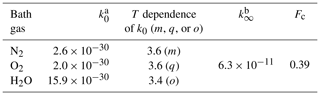a Units of cm6 molecule−2 s−1. b Units of cm3 molecule−1 s−1. Note that k is independent of temperature (n=0).

In the absence of experimental data on the reactions of HOONO with OH or on its photolysis, we follow the approach of Golden and Smith (2000) and set these equal to those for HO2NO2:

$\begin{array}{}\text{(R7)}& & \mathrm{HOONO}+\mathrm{OH}\to {\mathrm{H}}_{\mathrm{2}}\mathrm{O}+{\mathrm{NO}}_{\mathrm{3}},\text{(R8)}& & \mathrm{HOONO}+h\mathit{\nu }\to \mathrm{HO}+{\mathrm{NO}}_{\mathrm{2}}.\end{array}$

In Fig. 5, we illustrate the global impact (annual average) of H2O vapour on the rate coefficient. We plot the fractional reduction in k1 at the Earth's surface when setting ${x}_{{\mathrm{H}}_{\mathrm{2}}\mathrm{O}}$ to zero rather than using the EMAC global water-vapour fields. We focus on the boundary layer as the H2O concentration is largest here and decreases rapidly with altitude.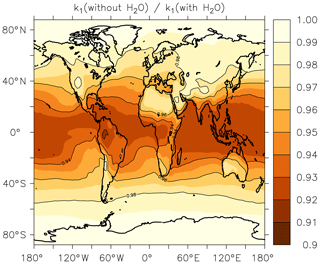Figure 5Annual-average effect of H2O on k1 expressed as the fractional change in the rate coefficient at the Earth's surface when setting the mole fraction of water vapour to zero in Eq. (15).

As expected, the greatest effect is found in warm, tropical regions where neglecting the impact of water vapour results in an average underestimation of the rate coefficient by up to ≈8 %. At higher or lower latitudes the effect is diminished and water vapour accounts for only 3 %–4 % of the overall rate coefficient at 40 N or S. The presence of water vapour does not impact on values of k1 above the boundary layer.

Our experimental data do not give insight into whether the H2O-induced enhancement in k1 is accompanied by a change in the branching ratio to favour either HNO3 or HOONO. However, as the formation of HOONO is favoured at high pressures (more effective collisional deactivation), it is possible that the HOONO yield may be enhanced relative to HNO3 in the presence of H2O. If this is the case, the increase in rate coefficient at high water-vapour levels (e.g. in the tropical lower troposphere) may be to some extent offset by the subsequent thermal dissociation of HOONO in these warm regions.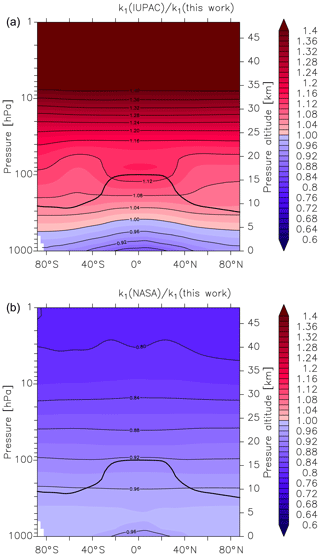Figure 6Global values of $\frac{{k}_{\mathrm{1}}^{\mathrm{IUPAC}}}{{k}_{\mathrm{1}}^{\mathrm{this}\phantom{\rule{0.25em}{0ex}}\mathrm{work}}}$ (a) and $\frac{{k}_{\mathrm{1}}^{\mathrm{NASA}}}{{k}_{\mathrm{1}}^{\mathrm{this}\phantom{\rule{0.25em}{0ex}}\mathrm{work}}}$ (b). k1 is the overall rate coefficient (both channels) for Reaction R1 calculated using the parameters from this work (${k}_{\mathrm{1}}^{\mathrm{this}\phantom{\rule{0.25em}{0ex}}\mathrm{work}}$) and those presently recommended by the IUPAC (${k}_{\mathrm{1}}^{\mathrm{IUPAC}}$) and NASA (${k}_{\mathrm{1}}^{\mathrm{NASA}}$) data evaluation panels. The black line represents the model tropopause.

As described by Amedro et al. (2019) (Fig. 1 of that paper), two expert panels (IUPAC, NASA) evaluating kinetic data for use in atmospheric modelling fail to reach consensus for the title reaction, with the preferred rate coefficients differing by as much as 50 % in the cold UTLS. For this reason, we have calculated values of $\frac{{k}_{\mathrm{1}}^{\mathrm{NASA}}}{{k}_{\mathrm{1}}^{\mathrm{this}\phantom{\rule{0.25em}{0ex}}\mathrm{work}}}$ and $\frac{{k}_{\mathrm{1}}^{\mathrm{IUPAC}}}{{k}_{\mathrm{1}}^{\mathrm{this}\phantom{\rule{0.25em}{0ex}}\mathrm{work}}}$ at different altitudes and latitudes (i.e. at different temperatures and pressures). We parameterized the rate coefficient using the expressions given in this work (Eq. 15, Table 3) and in the latest evaluations of IUPAC (k1 last evaluated in 2017; IUPAC, 2019) and NASA (last evaluation published in 2015; Burkholder et al., 2015). As displayed in Fig. 6, values of $\frac{{k}_{\mathrm{1}}^{\mathrm{NASA}}}{{k}_{\mathrm{1}}^{\mathrm{this}\phantom{\rule{0.25em}{0ex}}\mathrm{work}}}$ and $\frac{{k}_{\mathrm{1}}^{\mathrm{IUPAC}}}{{k}_{\mathrm{1}}^{\mathrm{this}\phantom{\rule{0.25em}{0ex}}\mathrm{work}}}$ vary greatly with pressure and temperature and thus altitude. The NASA recommendations are always slightly lower but in good agreement (≤10 %) for most of the troposphere, with larger differences (($\frac{{k}_{\mathrm{1}}^{\mathrm{NASA}}}{{k}_{\mathrm{1}}^{\mathrm{this}\phantom{\rule{0.25em}{0ex}}\mathrm{work}}}$) always <1) only observed in the lower and mid-stratosphere. At altitudes above ≈30 km the ratio decreases to ≈0.8. A comparison with the rate coefficient derived from the IUPAC parameterization shows that $\frac{{k}_{\mathrm{1}}^{\mathrm{IUPAC}}}{{k}_{\mathrm{1}}^{\mathrm{this}\phantom{\rule{0.25em}{0ex}}\mathrm{work}}}$ varies from ≈0.9 at the surface to ≈1.1 at the tropopause but increases to >1.3 at the low pressures and temperatures that reign at 30 km and above. At high altitudes (low pressure and temperature) the rate coefficients that the evaluation panels recommend are strongly biased by choice of the rate coefficient (and its temperature dependence) at the low-pressure limit. As discussed by Amedro et al. (2019) the available experimental data at low pressures and temperatures are not of sufficient accuracy to use as basis for recommendation of k0, and this is reflected in the highly divergent values of k1 under these conditions.

As mentioned above, the atmospheric HNO3∕NO2 ratio is expected to be highly sensitive to the rate coefficient k1, with an increase in k1 resulting in an increase in the HNO3∕NO2 ratio and vice versa. The HNO3∕NO2 ratio also depends on the concentration of OH, and thus the effect of using different values of k1 will be most apparent in regions where the greatest OH concentrations are found, i.e. at low latitudes. At higher latitudes, especially in winter months where solar insolation is weak and OH levels are relatively low, the HNO3∕NO2 ratio will also be impacted by night-time conversion of NO2 to N2O5 and finally, via heterogeneous hydrolysis, to HNO3. In Fig. 7 we plot zonally and yearly averaged model values of $\frac{{\mathrm{HNO}}_{\mathrm{3}}}{{\mathrm{NO}}_{\mathrm{2}}}\left(\mathrm{IUPAC}\right)/\frac{{\mathrm{HNO}}_{\mathrm{3}}}{{\mathrm{NO}}_{\mathrm{2}}}\left(\mathrm{this}\phantom{\rule{0.25em}{0ex}}\mathrm{work}\right)$ in the upper panel (Fig. 7a) and $\frac{{\mathrm{HNO}}_{\mathrm{3}}}{{\mathrm{NO}}_{\mathrm{2}}}\left(\mathrm{NASA}\right)/\frac{{\mathrm{HNO}}_{\mathrm{3}}}{{\mathrm{NO}}_{\mathrm{2}}}\left(\mathrm{this}\phantom{\rule{0.25em}{0ex}}\mathrm{work}\right)$ in the lower panel (Fig. 7b). Compared to the present parameterization of k1, the IUPAC evaluation returns HNO3∕NO2 ratios that are between 0.9 and 1 throughout most of the lower and free troposphere (up to ≈5 km) and larger HNO3∕NO2 ratios (factor of 1.1 to 1.15) above ≈10 km especially at the tropical tropopause. The divergence between the HNO3∕NO2 ratios increases as we move further into the stratosphere with $\frac{{\mathrm{HNO}}_{\mathrm{3}}}{{\mathrm{NO}}_{\mathrm{2}}}\left(\mathrm{IUPAC}\right)/\frac{{\mathrm{HNO}}_{\mathrm{3}}}{{\mathrm{NO}}_{\mathrm{2}}}\left(\mathrm{this}\phantom{\rule{0.25em}{0ex}}\mathrm{work}\right)$ values as large as 1.2 to 1.3 above 25 km. At the same time, NOx levels (NOx= NO +NO2) decrease by a factor of ≈0.95 (see Fig. S9). When we compare our parameterization with that of the NASA panel, the picture is largely reversed (lower panel, Fig. 7b). Again, we find reasonable agreement in the HNO3∕NO2 ratio in the lowermost atmosphere, but in this case lower values (0.8 to 0.9) in the lower stratosphere, which are accompanied by a factor of 1.06 change in NOx concentrations (Fig. S9). For both the NASA and IUPAC parameterizations, the largest differences in the HNO3∕NO2 ratio compared to the present study are found higher in the atmosphere. The modelling studies confirm the simple calculation of Amedro et al. (2019; see Fig. 1 of their paper), showing that the IUPAC and NASA parameterizations result in very different values of k1 in some parts of the atmosphere, and they will result in divergent predictions of partitioning of reactive nitrogen between NOx and NOy. Use of the parameterization based on the present dataset lies roughly between the two evaluations, with best agreement observed with NASA for the lower atmosphere. However, as previous laboratory studies had not identified the important role of H2O in the title reaction, which could therefore not be incorporated in either of the previous parameterizations, any agreement at better than the 10 % level is fortuitous, reflecting random cancelling of systematic bias.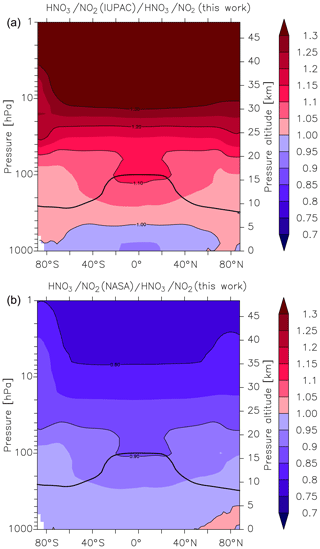Figure 7Effect of different parameterizations of k1 on the global (zonal and yearly averaged) HNO3 to NO2 ratio. (a) $\frac{{\mathrm{HNO}}_{\mathrm{3}}}{{\mathrm{NO}}_{\mathrm{2}}}\left(\mathrm{IUPAC}\right)/\frac{{\mathrm{HNO}}_{\mathrm{3}}}{{\mathrm{NO}}_{\mathrm{2}}}\left(\mathrm{this}\phantom{\rule{0.25em}{0ex}}\mathrm{work}\right)$, (b) $\frac{{\mathrm{HNO}}_{\mathrm{3}}}{{\mathrm{NO}}_{\mathrm{2}}}\left(\mathrm{NASA}\right)/\frac{{\mathrm{HNO}}_{\mathrm{3}}}{{\mathrm{NO}}_{\mathrm{2}}}\left(\mathrm{this}\phantom{\rule{0.25em}{0ex}}\mathrm{work}\right)$. The black line represents the model tropopause.

As reaction with OH is the predominant sink for most atmospheric trace gases, its concentration largely defines the oxidizing power of the atmosphere (Lelieveld et al., 2004, 2008, 2016) and even changes of a few percent in its concentration are significant. An increase in the rate coefficient of the title reaction will reduce the atmospheric abundance of this centrally important radical. In Fig. S10 we illustrate the impact of using the parameterization of k1 from the present study compared to the IUPAC and NASA recommendations. The upper panel in Fig. S10 plots the ratio of OH concentrations obtained when using the IUPAC parameterization and that from the present study, OH(IUPAC)/OH(this work). Throughout the troposphere OH(IUPAC)/OH(this work) deviates by only a few percent, with a value of 1.02 at the surface and 0.96 at the tropical tropopause. OH(NASA)/OH(this work) is also 1.02 at the surface but increases to 1.04 at the tropical tropopause as the NASA-derived value of k1 is lower at the temperatures and pressures encountered in this part of the atmosphere. The weak effect of changing k1 on OH at the surface reflects the fact that many reactions apart from that with NO2 contribute to the overall sink term for OH in the lower troposphere.

Although our experiments do not give insight into the branching between formation of HOONO and HNO3 in the title reaction, previous work predicts a significant yield of HOONO especially at low temperatures (see Fig. S8). As the lifetime of HOONO with respect to re-dissociation to reactants is short at, for example, boundary layer temperatures (≈1 s at 298 K and 1 bar pressure), its formation may be seen as an effective reduction in the rate coefficient for OH +NO2 (Golden and Smith, 2000). However, its lifetime increases to several days at temperature and pressure conditions typical of the tropical tropopause (100 mbar, 220 K). As HOONO formation and loss are now parameterized (see above) in EMAC, we can explore its potential contribution to odd-nitrogen species in the atmosphere. The reaction between OH and NO2 to form HOONO converts short-lived HOx (HOx= OH +HO2) and NOx (NOx= NO +NO2) into a longer-lived “reservoir” species, and in this sense it is similar to the reaction between HO2 and NO2 to form HO2NO2:

$\begin{array}{}\text{(R9)}& {\mathrm{HO}}_{\mathrm{2}}+{\mathrm{NO}}_{\mathrm{2}}+\mathrm{M}\to {\mathrm{HO}}_{\mathrm{2}}{\mathrm{NO}}_{\mathrm{2}}+\mathrm{M},\end{array}$

which is also thermally unstable, dissociating to reform HO2 and NO2. Unlike HOONO, for which there are no atmospheric measurements, much effort has been made to measure concentrations of HO2NO2 in colder regions of the atmosphere, and it is considered an important component of the NOy budget at high altitudes (Nault et al., 2016). We therefore compared EMAC predictions of HOONO concentrations with those of HO2NO2. The results are displayed in Fig. 8, in which we plot the zonally averaged HOONO∕HO2NO2 ratio. Immediately apparent from Fig. 8 is that, compared to HO2NO2, HOONO is a minor component of NOy in the warm, lower atmosphere. This reflects the difference in the thermal decomposition rate constant of the two trace gases, i.e. that of HO2NO2 being $\approx \mathrm{4}×{\mathrm{10}}^{-\mathrm{5}}$ s−1 in, for example, the middle troposphere at 400 mbar and 250 K, whereas HOONO decomposes a 10 times faster so that its lifetime is only ≈1000 s. In the UTLS region, the ratio increases further (HO2NO2 is a factor of 50 more long lived with respect to thermal decomposition at 100 mbar and 220 K), but the lifetimes of both gases under these conditions are sufficiently long that their concentrations are largely determined by their production rates and their losses due to photolysis and reaction with OH. The maximum ratio of HOONO to HO2NO2 is found at the tropical tropopause, where concentrations become comparable. As the modelled loss processes of HOONO and HO2NO2 (rate constants for photolysis and reaction with OH) are assumed to be identical, the occurrence of the maximum HOONO to HO2NO2 ratio at the tropical tropopause is related to the ratio of the (temperature-dependent) rate coefficients responsible for their formation (at 220 K and 100 mbar this favours HOONO formation by a factor of ≈2) and the model OH∕HO2 ratio. Whilst this result indicates that HOONO could be an important reservoir of NOx under certain conditions, we must bear in mind that there is great uncertainty associated not only with the branching ratio to HOONO formation in Reaction (R1b) but also with its loss processes (reaction with OH, photolysis), which remain unexplored experimentally. OH reacts with HO2NO2 via H abstraction from the H−OO group (IUPAC, 2019), and a similar mechanism is likely for HOONO. As the H−OO bond strength is likely to be greater in HOONO than in HO2NO2 (larger electron density around the peroxy bond), we may expect the rate coefficient to be lower for HOONO. A significantly lower rate coefficient for reaction with OH (or photolysis rate constant) could greatly increase the abundance of HOONO. If this were the case, airborne instruments that measure NOx would likely also measure some fraction of HOONO following its rapid decomposition in warm inlet lines, as has been observed for HO2NO2 and CH3O2NO2 (Nault et al., 2015; Silvern et al., 2018). Clearly, more experimental or theoretical data that better constrain the yield of HOONO and its atmospheric loss processes as well as atmospheric measurements are necessary in order to improve our understanding of the role of the reaction between OH and NO2 throughout the atmosphere.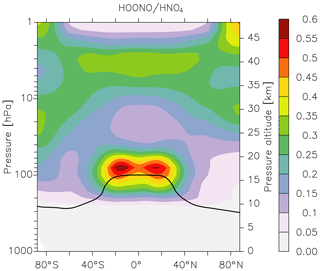Figure 8Model (EMAC) ratio of HOONO (formed in the reaction of NO2 with OH) to HO2NO2 (formed in the reaction of NO2 with HO2) calculated using the present parameterization of k1 and equating the (unknown) rate coefficients for loss of HOONO via reaction with OH or photolysis to those of HO2NO2. The black line represents the model tropopause.

4 Conclusions

We have made very precise and accurate measurements for the overall rate coefficient, k1, of the reaction between OH and NO2, which is of critical importance in atmospheric chemistry. Our experiments demonstrate clearly that the presence of H2O increases significantly the overall rate coefficient (k1) of the reaction between OH and NO2. H2O is found to be a more efficient collisional quencher (by a factor of ≈6) of the initially formed HO–NO2 association complex than N2 and a factor of ≈8 more efficient than O2. A new parameterization of the rate coefficient for the title reaction that considers the roles of N2, O2, and H2O as third-body quenchers (also using data from our previous paper; Amedro et al., 2019) has been incorporated into a global chemistry transport model to assess its impact on, for example, the HNO3∕NO2 ratio as well as NOx and OH levels. Compared to existing evaluations of the kinetic data, use of the new parameters will result in significant changes (5 %–10 %) in the partitioning of NOx and NOy, the direction of the bias depending on which evaluation is used as reference and on region of the atmosphere. This work highlights the continuing importance of obtaining accurate laboratory kinetic data for those reactions that are central to our understanding of atmospheric chemistry and which provide anchor-points in chemical transport models.

Though the result is associated with great uncertainty owing to missing kinetic parameters for HOONO, the global model predicts the presence of HOONO in concentrations similar to those of HO2NO2 at the tropical tropopause. The present dataset addresses only the overall rate coefficient (k1). Detailed experimental studies of the formation of HOONO (e.g. its yield at various temperatures and in the presence of H2O) and on the fate of HOONO (OH kinetics, photolysis) are required to better assess its role as an NOx and HOx reservoir in cold parts of the atmosphere.

Data availability

The rate coefficients measured during this experimental study are listed in Table 1.

Supplement

Author contributions

The experiments were carried out by DA, AJCB, and MB. The data analysis and preparation of the paper were performed by DA, with assistance from JL and JNC. The global modelling was performed by AP.

Competing interests

The authors declare that they have no conflict of interest.

Financial support

The article processing charges for this open-access publication were covered by the Max Planck Society.

Review statement

This paper was edited by Rainer Volkamer and reviewed by three anonymous referees.

References

Allodi, M. A., Dunn, M. E., Livada, J., Kirschner, K. N., and Shields, G. C.: Do hydroxyl radical-water clusters, OH(H2O)(n), n=1–5, exist in the atmosphere?, J. Phys. Chem. A, 110, 13283-13289, https://doi.org/10.1021/jp064468l, 2006.

Amedro, D., Bunkan, A. J. C., Berasategui, M., and Crowley, J. N.: Kinetics of the OH+ NO2 reaction: rate coefficients (217–333 K, 16–1200 mbar) and fall-off parameters for N2 and O2 bath gases, Atmos. Chem. Phys., 19, 10643–10657, https://doi.org/10.5194/acp-19-10643-2019, 2019.

Anastasi, C., and Smith, I. W. M.: Rate measurements of reactions of OH by resonance absorption. Part 5. – Rate constants for OH +NO2 ($+M\right)\to {\mathrm{HNO}}_{\mathrm{3}}\left(+M\right)$ over a wide range of temperature and pressure, J. Chem. Soc. Faraday Transact., 72, 1459–1468, https://doi.org/10.1039/f29767201459, 1976.

Anderson, J. G., Margitan, J. J., and Kaufman, F.: Gas-phase recombination of OH with NO and NO2, J. Chem. Phys., 60, 3310–3317, 1974.

Anderson, L. G.: Absolute rate constants for the reaction of OH with NO2 in N2 and He from 225 to 389 K, J. Phys. Chem., 84, 2152–2155, 1980.

Burke, M. P. and Song, R.: Evaluating mixture rules for multi-component pressure dependence: H +O2($+M\right)={\mathrm{HO}}_{\mathrm{2}}\left(+M\right)$, Proc. Combust. Inst., 36, 245–253, https://doi.org/10.1016/j.proci.2016.06.068, 2017.

Burkholder, J. B., Sander, S. P., Abbatt, J., Barker, J. R., Huie, R. E., Kolb, C. E., Kurylo, M. J., Orkin, V. L., Wilmouth, D. M., and Wine, P. H.: Chemical Kinetics and Photochemical Data for Use in Atmospheric Studies, Evaluation No. 18, JPL Publication 15-10, Jet Propulsion Laboratory, Pasadena, available at: http://jpldataeval.jpl.nasa.gov (last access: November 2019), 2015.

Buszek, R. J., Francisco, J. S., and Anglada, J. M.: Water effects on atmospheric reactions, Int. Rev. Phys. Chem., 30, 335–369, https://doi.org/10.1080/0144235X.2011.634128, 2011.

Cantrell, C. A., Zimmer, A., and Tyndall, G. S.: Absorption cross sections for water vapor from 183 to 193 nm, Geophys. Res. Lett., 24, 2195–2198, 1997.

Cobos, C. J. and Troe, J.: Prediction of reduced falloff curves for recombination reactions at low temperatures, Z. Phys. Chem., 217, 1031–1044, 2003.

Deckert, R., Jöckel, P., Grewe, V., Gottschaldt, K.-D., and Hoor, P.: A quasi chemistry-transport model mode for EMAC, Geosci. Model Dev., 4, 195–206, https://doi.org/10.5194/gmd-4-195-2011, 2011.

de Meij, A., Pozzer, A., Pringle, K. J., Tost, H., and Lelieveld, J.: EMAC model evaluation and analysis of atmospheric aerosol properties and distribution with a focus on the Mediterranean region, Atmos. Res., 114, 38–69, 2012.

D'Ottone, L., Campuzano-Jost, P., Bauer, D., and Hynes, A. J.: A pulsed laser photolysis-pulsed laser induced fluorescence study of the kinetics of the gas-phase reaction of OH with NO2, J. Phys. Chem. A, 105, 10538–10543, 2001.

D'Ottone, L., Bauer, D., Campuzano-Jost, P., Fardy, M., and Hynes, A. J.: Kinetic and mechanistic studies of the recombination of OH with NO2: Vibrational deactivation, isotopic scrambling and product isomer branching ratios, Faraday Discuss., 130, 111–123, 2005.

Dulitz, K., Amedro, D., Dillon, T. J., Pozzer, A., and Crowley, J. N.: Temperature-(208–318 K) and pressure-(18–696 Torr) dependent rate coefficients for the reaction between OH and HNO3, Atmos. Chem. Phys., 18, 2381–2394, https://doi.org/10.5194/acp-18-2381-2018, 2018.

Elshorbany, Y. F., Crutzen, P. J., Steil, B., Pozzer, A., Tost, H., and Lelieveld, J.: Global and regional impacts of HONO on the chemical composition of clouds and aerosols, Atmos. Chem. Phys., 14, 1167–1184, https://doi.org/10.5194/acp-14-1167-2014, 2014.

Erler, K., Field, D., Zellner, R., and Smith, I. W. M.: Recombination reaction between hydroxyl radicals and nitrogen-dioxide: OH +NO2+M (= He, CO2) in temperature range 213–300 K, Phys. Chem. Chem. Phys., 81, 22–26, https://doi.org/10.1002/bbpc.19770810107, 1977.

Fernandes, R. X., Luther, K., Troe, J., and Ushakov, V. G.: Experimental and modelling study of the recombination reaction H +O2 ($+M\right)\to {\mathrm{HO}}_{\mathrm{2}}$ (+M) between 300 and 900 K, 1.5 and 950 bar, and in the bath gases M= He, Ar, and N2, Phys. Chem. Chem. Phys., 10, 4313–4321, https://doi.org/10.1039/b804553d, 2008.

Getzinger, R. W. and Blair, L. S.: Recombination in the hydrogen-oxygen reaction: A shock tube study with nitrogen and water vapour as third bodies, Combust. Flame, 13, 271–284, https://doi.org/10.1016/0010-2180(69)90005-4, 1969.

Gilbert, R. G., Luther, K., and Troe, J.: Theory of thermal unimolecular reactions in the fall-off range. 2. weak collision rate constants, Phys. Chem. Chem. Phys., 87, 169–177, 1983.

Glänzer, K. and Troe, J.: Thermal Decomposition of Nitrocompounds in Shock Waves. IV: Decomposition of Nitric Acid, Bericht. Bunsengesell. Phys. Chem., 78, 71–76, 1974.

Golden, D. M., and Smith, G. P.: Reaction of OH +NO2+M: A new view, J. Phys. Chem. A, 104, 3991–3997, 2000.

Golden, D. M., Barker, J. R., and Lohr, L. L.: Master equation models for the pressure- and temperature-dependant reactions HO +NO2→HONO2 and HO +NO2 HOONO, J. Phys. Chem. A, 107, 11057–11071, 2003.

Hippler, H., Krasteva, N., Nasterlack, S., and Striebel, F.: Reaction of OH +NO2: High pressure experiments and falloff analysis, J. Phys. Chem. A, 110, 6781–6788, 2006.

IUPAC: Task Group on Atmospheric Chemical Kinetic Data Evaluation, edited by: Ammann, M., Cox, R. A., Crowley, J. N., Herrmann, H., Jenkin, M. E., McNeill, V. F., Mellouki, A., Rossi, M. J., Troe, J., and Wallington, T. J., available at: http://iupac.pole-ether.fr/index.html, last access: November 2019.

Jeuken, A. B. M., Siegmund, P. C., Heijboer, L. C., Feichter, J., and Bengtsson, L.: On the potential of assimilating meteorological analyses in a global climate model for the purpose of model validation, J. Geophys. Res.-Atmos., 101, 16939–16950, 1996.

Jöckel, P., Tost, H., Pozzer, A., Brühl, C., Buchholz, J., Ganzeveld, L., Hoor, P., Kerkweg, A., Lawrence, M. G., Sander, R., Steil, B., Stiller, G., Tanarhte, M., Taraborrelli, D., van Aardenne, J., and Lelieveld, J.: The atmospheric chemistry general circulation model ECHAM5/MESSy1: consistent simulation of ozone from the surface to the mesosphere, Atmos. Chem. Phys., 6, 5067–5104, https://doi.org/10.5194/acp-6-5067-2006, 2006.

Jöckel, P., Kerkweg, A., Pozzer, A., Sander, R., Tost, H., Riede, H., Baumgaertner, A., Gromov, S., and Kern, B.: Development cycle 2 of the Modular Earth Submodel System (MESSy2), Geosci. Model Dev., 3, 717–752, https://doi.org/10.5194/gmd-3-717-2010, 2010.

Kircher, C. C. and Sander, S. P.: Kinetics and mechanism of HO2 and DO2 disproportionations, J. Phys. Chem., 88, 2082–2091, 1984.

Lelieveld, J., Dentener, F. J., Peters, W., and Krol, M. C.: On the role of hydroxyl radicals in the self-cleansing capacity of the troposphere, Atmos. Chem. Phys., 4, 2337–2344, https://doi.org/10.5194/acp-4-2337-2004, 2004.

Lelieveld, J., Butler, T. M., Crowley, J. N., Dillon, T. J., Fischer, H., Ganzeveld, L., Harder, H., Lawrence, M. G., Martinez, M., Taraborrelli, D., and Williams, J.: Atmospheric oxidation capacity sustained by a tropical forest, Nature, 452, 737–740, 2008.

Lelieveld, J., Gromov, S., Pozzer, A., and Taraborrelli, D.: Global tropospheric hydroxyl distribution, budget and reactivity, Atmos. Chem. Phys., 16, 12477–12493, https://doi.org/10.5194/acp-16-12477-2016, 2016.

Lii, R.-R., Sauer, M. C., and Gordon, S.: Temperature dependence of the gas-phase self-reaction of HO2 in the presence of H2O, J. Phys. Chem., 85, 2833–2834, 1981.

Michael, J. V., Su, M. C., Sutherland, J. W., Carroll, J. J., and Wagner, A. F.: Rate constants for H +O2+$M\to {\mathrm{HO}}_{\mathrm{2}}+M$ in seven bath gases, J. Phys. Chem. A, 106, 5297–5313, https://doi.org/10.1021/jp020229w, 2002.

Mollner, A. K., Valluvadasan, S., Feng, L., Sprague, M. K., Okumura, M., Milligan, D. B., Bloss, W. J., Sander, S. P., Martien, P. T., Harley, R. A., McCoy, A. B., and Carter, W. P. L.: Rate of gas phase association of hydroxyl radical and nitrogen dioxide, Science, 330, 646–649, https://doi.org/10.1126/science.1193030, 2010.

Morley, C. and Smith, I. W. M.: Rate measurements of reactions of OH by resonance absorption. 1. Reactions of OH with NO2 and NO, J. Chem. Soc.-Faraday Trans. II, 68, 1016–1030, https://doi.org/10.1039/f29726801016, 1972.

Nault, B. A., Garland, C., Pusede, S. E., Wooldridge, P. J., Ullmann, K., Hall, S. R., and Cohen, R. C.: Measurements of CH3O2NO2 in the upper troposphere, Atmos. Meas. Tech., 8, 987–997, https://doi.org/10.5194/amt-8-987-2015, 2015.

Nault, B. A., Garland, C., Wooldridge, P. J., Brune, W. H., Campuzano-Jost, P., Crounse, J. D., Day, D. A., Dibb, J., Hall, S. R., Huey, L. G., Jimenez, J. L., Liu, X. X., Mao, J. Q., Mikoviny, T., Peischl, J., Pollack, I. B., Ren, X. R., Ryerson, T. B., Scheuer, E., Ullmann, K., Wennberg, P. O., Wisthaler, A., Zhang, L., and Cohen, R. C.: Observational Constraints on the Oxidation of NOx in the Upper Troposphere, J. Phys. Chem. A, 120, 1468–1478, https://doi.org/10.1021/acs.jpca.5b07824, 2016.

Newsome, B. and Evans, M.: Impact of uncertainties in inorganic chemical rate constants on tropospheric composition and ozone radiative forcing, Atmos. Chem. Phys., 17, 14333–14352, https://doi.org/10.5194/acp-17-14333-2017, 2017.

Pozzer, A., Pollmann, J., Taraborrelli, D., Jöckel, P., Helmig, D., Tans, P., Hueber, J., and Lelieveld, J.: Observed and simulated global distribution and budget of atmospheric C2C5 alkanes, Atmos. Chem. Phys., 10, 4403–4422, https://doi.org/10.5194/acp-10-4403-2010, 2010.

Roeckner, E., Brokopf, R., Esch, M., Giorgetta, M., Hagemann, S., Kornblueh, L., Manzini, E., Schlese, U., and Schulzweida, U.: Sensitivity of simulated climate to horizontal and vertical resolution in the ECHAM5 atmosphere model, J. Climate, 19, 3771–3791, 2006.

Sadanaga, Y., Kondo, S., Hashimoto, K., and Kajii, Y.: Measurement of the rate coefficient for the OH +NO2 reaction under the atmospheric pressure: Its humidity dependence, Chem. Phys. Lett., 419, 474–478, 2006.

Sander, R., Baumgaertner, A., Cabrera-Perez, D., Frank, F., Gromov, S., Grooss, J. U., Harder, H., Huijnen, V., Jockel, P., Karydis, V. A., Niemeyer, K. E., Pozzer, A., Hella, R. B., Schultz, M. G., Taraborrelli, D., and Tauer, S.: The community atmospheric chemistry box model CAABA/MECCA-4.0, Geosci. Model Dev., 12, 1365–1385, https://doi.org/10.5194/gmd-12-1365-2019, 2019.

Shao, J., Choudhary, R., Susa, A., Davidson, D. F., and Hanson, R. K.: Shock tube study of the rate constants for H +O2+MHO2+M (M= Ar, H2O, CO2, N2) at elevated pressures, Proc. Combust. Inst., 37, 145–152, https://doi.org/10.1016/j.proci.2018.05.077, 2019.

Silvern, R. F., Jacob, D. J., Travis, K. R., Sherwen, T., Evans, M. J., Cohen, R. C., Laughner, J. L., Hall, S. R., Ullmann, K., Crounse, J. D., Wennberg, P. O., Peischl, J., and Pollack, I. B.: Observed NO∕NO2 ratios in the upper troposphere imply errors in NO-NO2-O3 cycling kinetics or an unaccounted NOx reservoir, Geophys. Res. Lett., 45, 4466–4474, https://doi.org/10.1029/2018gl077728, 2018.

Simonaitis, R. and Heicklen, J.: The reaction of OH wth NO2 and the deactivation of O(1D) by CO, Int. J. Chem. Kinet., IV, 529–540, 1972.

Smith, I. W. and Williams, M. D.: Vibrational-relaxation of OH(v=1) and OD(v=1) By HNO3, DNO3, H2O, NO and NO2, J. Chem. Soc. Faraday Transact. 2, 81, 1849–1860, 1985.

Tao, F. M., Higgins, K., Klemperer, W., and Nelson, D. D.: Structure, binding energy, and equilibrium constant of the nitric acid-water complex, Geophys. Res. Lett., 23, 1797–1800, 1996.

Thomsen, D. L., Kurten, T., Jorgensen, S., Wallington, T. J., Baggesen, S. B., Aalling, C., and Kjaergaard, H. G.: On the possible catalysis by single water molecules of gas-phase hydrogen abstraction reactions by OH radicals, Phys. Chem. Chem. Phys., 14, 12992–12999, https://doi.org/10.1039/c2cp40795g, 2012.

Troe, J.: Mixture Rules in Thermal Unimolecular Reactions, Bericht. Bunsengesell. Phys. Chem., 84, 829–834, https://doi.org/10.1002/bbpc.19800840902, 1980.

Troe, J.: Theory of thermal unimolecular reactions in the fall-off range 1. Strong collision rate constants, Phys. Chem. Chem. Phys., 87, 161–169, 1983.

Troe, J.: Analysis of the temperature and pressure dependence of the reaction HO +NO2+$M\to {\mathrm{HONO}}_{\mathrm{2}}+M$, Int. J. Chem. Kinet., 33, 878–889, 2001.

Troe, J.: Toward a Quantitative Analysis of Association Reactions in the Atmosphere, Chem. Rev., 103, 4565–4576, https://doi.org/10.1021/cr020514b, 2003.

Troe, J.: Refined representation of falloff curves for the reaction HO +${\mathrm{NO}}_{\mathrm{2}}+{\mathrm{N}}_{\mathrm{2}}\to \left({\mathrm{HONO}}_{\mathrm{2}}$, HOONO) +N2, J. Phys. Chem. A, 116, 6387–6393, https://doi.org/10.1021/jp212095n, 2012.

Troe, J. and Ushakov, V. G.: Revisiting falloff curves of thermal unimolecular reactions, J. Chem. Phys., 135, 054304, https://doi.org/10.1063/1.3615542, 2011.

Vandaele, A. C., Hermans, C., Fally, S., Carleer, M., Colin, R., Merienne, M. F., Jenouvrier, A., and Coquart, B.: High-resolution Fourier transform measurement of the NO2 visible and near-infrared absorption cross sections: Temperature and pressure effects, J. Geophys. Res.-Atmos., 107, 4348, https://doi.org/10.1029/2001JD000971, 2002.

Westenberg, A. A. and Dehaas, N.: Rate measurements on OH + NO +M and OH +NO2+M, J. Chem. Phys., 57, 5375–5378, https://doi.org/10.1063/1.1678234, 1972.

Wine, P. H., Kreutter, N. M., and Ravishankara, A. R.: Flash photolysis-resonance fluorescence kinetics study of the reaction OH +${\mathrm{NO}}_{\mathrm{2}}+M\to {\mathrm{HNO}}_{\mathrm{3}}+M$, J. Phys. Chem., 83, 3191–3195, 1979.

Wollenhaupt, M., Carl, S. A., Horowitz, A., and Crowley, J. N.: Rate coefficients for reaction of OH with acetone between 202 and 395 K, J. Phys. Chem., 104, 2695–2705, https://doi.org/10.1021/jp993738f, 2000.

Yoon, J. and Pozzer, A.: Model-simulated trend of surface carbon monoxide for the 2001–2010 decade, Atmos. Chem. Phys., 14, 10465–10482, https://doi.org/10.5194/acp-14-10465-2014, 2014.